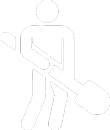# ORDER CALCULATOR

## Please choose the square/rectangular calculator or Circle calculator form below. The cubic yard total will be automatically rounded up ( example: 1.7 = 2)

Calculate Here